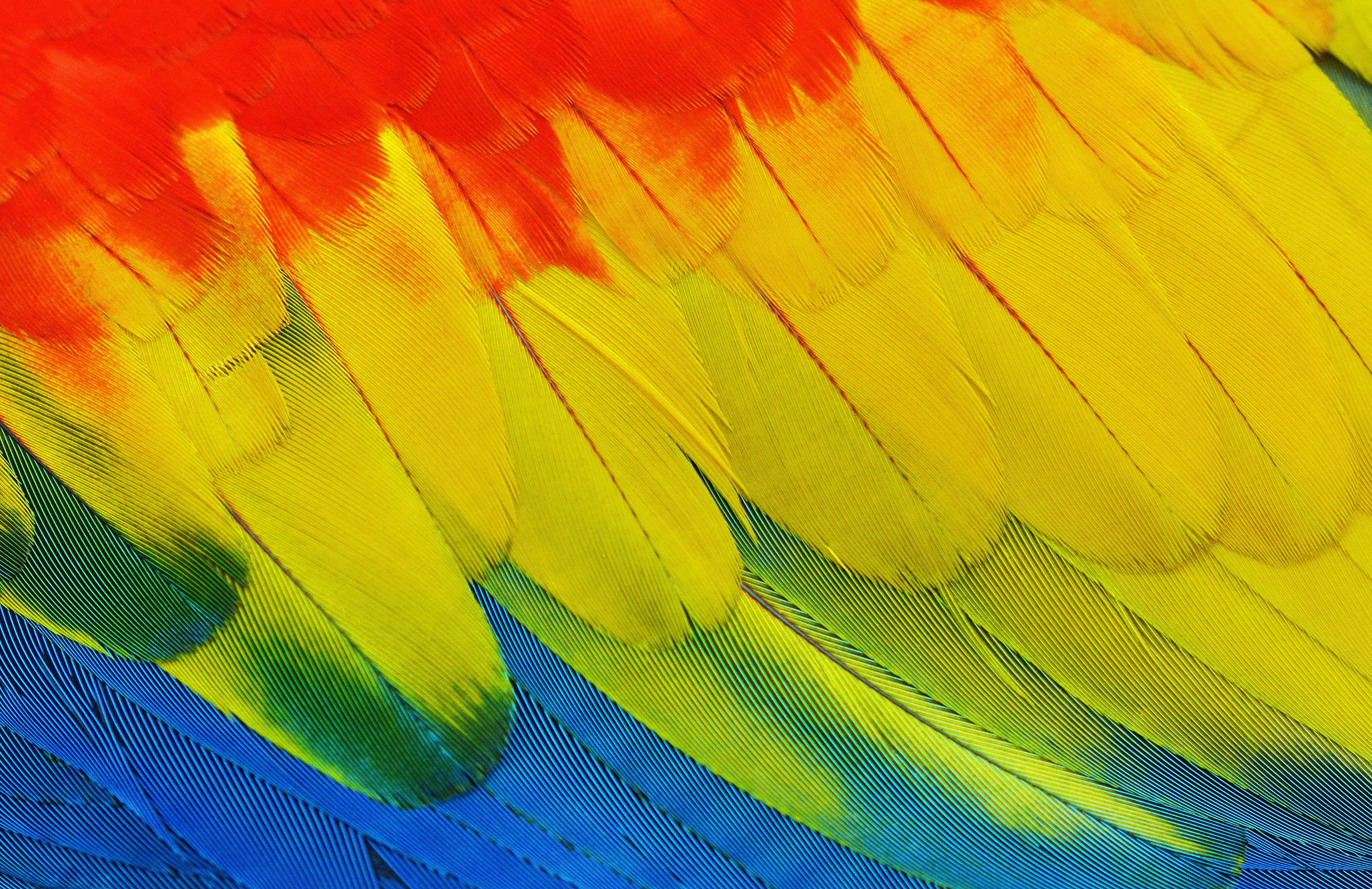## Super-Fast Color Transfer Algorithm

Published on in Algorithms## The Algorithm

The algorithm that I'm going to mention is based on the following paper:

Color transfer between images by E. Reinhard

In this paper, Reinhard and colleagues demonstrate that by utilizing the Lab* color space and the mean and standard deviation of each L*, a*, and b* channel, respectively, that the color can be transferred between two images.

The algorithm performs the following:

1. Input a source and a target image. The source image contains the color space that you want your target image to mimic.
2. Convert both the source and the target image to the Lab* color space. The Lab* color space models perceptual uniformity, where a small change in an amount of color value should also produce a relatively equal change in color importance. The Lab* color space does a substantially better job mimicking how humans interpret color than the standard RGB color space, and as you’ll see, works very well for color transfer.
3. Split the channels for both the source and target.
4. Compute the mean and standard deviation of each of the Lab* channels for the source and target images.
5. Subtract the mean of the Lab* channels of the target image from target channels.
6. Scale the target channels by the ratio of the standard deviation of the target divided by the standard deviation of the source, multiplied by the target channels.
7. Add in the means of the Lab* channels for the source.
8. Clip any values that fall outside the range [0, 255].
9. Merge the channels back together.
10. Convert back to the RGB color space from the Lab* space.

## The Code

Make sure to have OpenCV for Python installed before you run the code.

## The Results

#### Image to transfer colors from:#### Image to transfer colors onto:#### The final image:## TODO:

Make a Flask webserver and convert this code into a REST API.